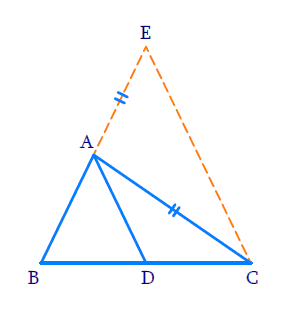# Ex. 6.6 Q9 Triangles Solution - NCERT Maths Class 10

Go back to  'Ex.6.6'

## Question

In Fig.below, $$D$$ is a point on side $$BC$$ of $$\Delta ABC$$ such that \begin{align}\frac{{BD}}{{CD}} = \frac{{BA}}{{CA}}\end{align}. Prove that $$AD$$ is the bisector of $$\angle BAC$$.Video Solution
Triangles
Ex 6.6 | Question 9

## Text Solution

#### Reasoning:

(i) As we know that in an isosceles triangle, the angles opposite to equal sides are equal.

(ii) Converse of BPT

#### Steps:

Extended $$BA$$ to $$E$$ such that $$AE = AC$$ and join $$CE.$$

In $$\Delta AEC$$

\begin{align}AE &= AC \\ \Rightarrow \angle ACE &= \angle AEC \;\;\dots\left(\text{i} \right) \end{align}

It is given that

\begin{align} \frac{{BD}}{{CD}} & = \frac{{BA}}{{CA}}\\ \frac{{BD}}{{CD}} & = \frac{{BA}}{{AE}} \;\;\cdots \left( \text{ii} \right) \\ & \left(\because {AC = AE} \right) \end{align}

In $$\Delta ABD$$ and $$\Delta EBC$$

\begin{align} &AD||EC\,\,\left( {{\text{Converse of BPT}}} \right)\\\\ & \Rightarrow \angle BAD = \angle BEC \cdots \left( \text{iii} \right) \\ &\quad \left( \text{Corresponding angles} \right) \\ & \qquad \qquad\text{and} \, \\&\quad\angle DAC = \angle ACE \cdots \left( \text{iv} \right) \\ &\quad \left( \text{Alternative Angles} \right) \end{align}

From $$\rm(i)$$, $$\rm(iii)$$ and $$\rm (iv)$$

\begin{align} &\angle BAD = \angle DAC\\ & \Rightarrow AD\,\text{is the bisector of}\;\angle BAC \end{align}

Learn from the best math teachers and top your exams

• Live one on one classroom and doubt clearing
• Practice worksheets in and after class for conceptual clarity
• Personalized curriculum to keep up with school